# 《pandas数据分析》（一）——数据基础操作

1. 第一部分：我们获得一个要分析的数据，我要学会如何加载数据，查看数据，然后学习Pandas的一些基础操作，最后开始尝试探索性的数据分析。
2. 第二部分：当我们可以比较熟练的操作数据并认识这个数据之后，我们需要开始数据清洗以及重构，将原始数据变为一个可用好用的数据，为之后放入模型做准备
3. 第三部分：我们根据任务需求不同，要考虑建立什么模型，我们使用流行的sklearn库，建立模型。对于一个模型的好坏，我们是需要评估的，之后我们会评估我们的模型，对模型做优化。

```Python

# 写入代码

import pandas as pd import numpy as np ```

【提示】如果加载失败，学会如何在你的python环境下安装numpy和pandas这两个库

(1) 使用相对路径载入数据
(2) 使用绝对路径载入数据

```Python

# 写入代码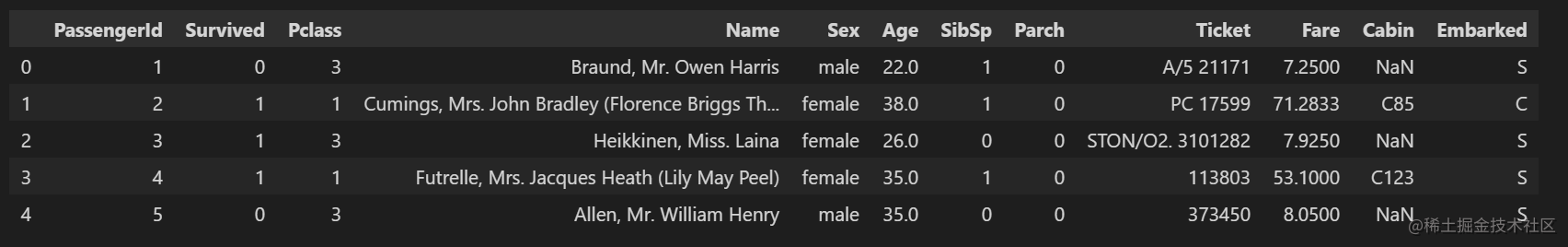【提示】相对路径载入报错时，尝试使用os.getcwd()查看当前工作目录。
【总结】加载的数据是所有工作的第一步，我们的工作会接触到不同的数据格式（eg:.csv;.tsv;.xlsx）,但是加载的方法和思路都是一样的，在以后工作和做项目的过程中，遇到之前没有碰到的问题，要多多查资料，使用googel，了解业务逻辑，明白输入和输出是什么。

```python

# 设置chunksize参数，来控制每次迭代数据的大小

chunker = pd.read_csv("train.csv",chunksize=1000) for piece in chunker: print(type(piece)) # print(len(piece)) #891 ```

【思考】什么是逐块读取？为什么要逐块读取呢？

#### 1.1.4 任务四：将表头改成中文，索引改为乘客ID （对于某些英文资料，我们可以通过翻译来更直观的熟悉我们的数据）

PassengerId => 乘客ID
Survived => 是否幸存
Pclass => 乘客等级(1/2/3等舱位)
Name => 乘客姓名
Sex => 性别
Age => 年龄
SibSp => 堂兄弟/妹个数
Parch => 父母与小孩个数
Ticket => 船票信息
Fare => 票价
Cabin => 客舱
Embarked => 登船港口

```Python

# 写入代码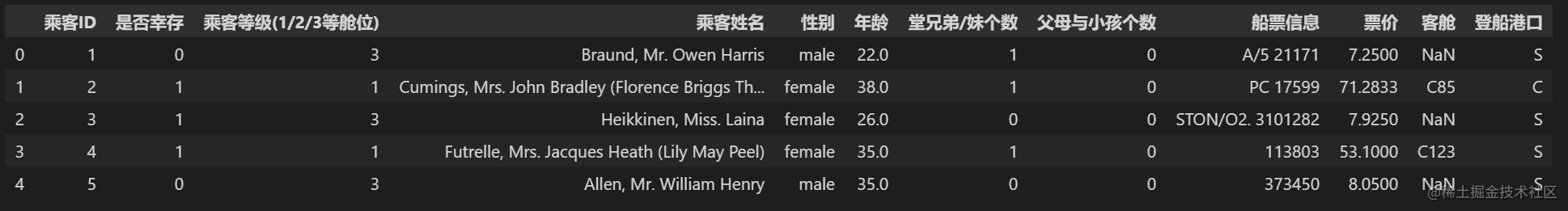【思考】所谓将表头改为中文其中一个思路是：将英文列名表头替换成中文。还有其他的方法吗？

```Python

# 查看每列的类型,dataframe的属性dtypes可以返回表示每列数据名称及类型的列表：

print(data.dtypes) ```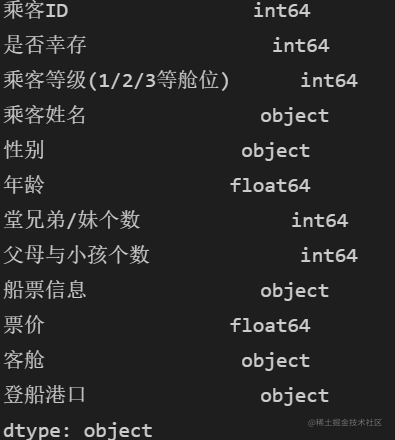```Python

# 使用：dataframe.describe()即可查看每列数据的

''' （1）总行数统计count （2）平均值mean （3）标准差std （4）最小值min （5）25%分位值“25%” （6）50%分位值“50%” （7）75%分位值“75%” （8）最大值max ''' data.describe() ```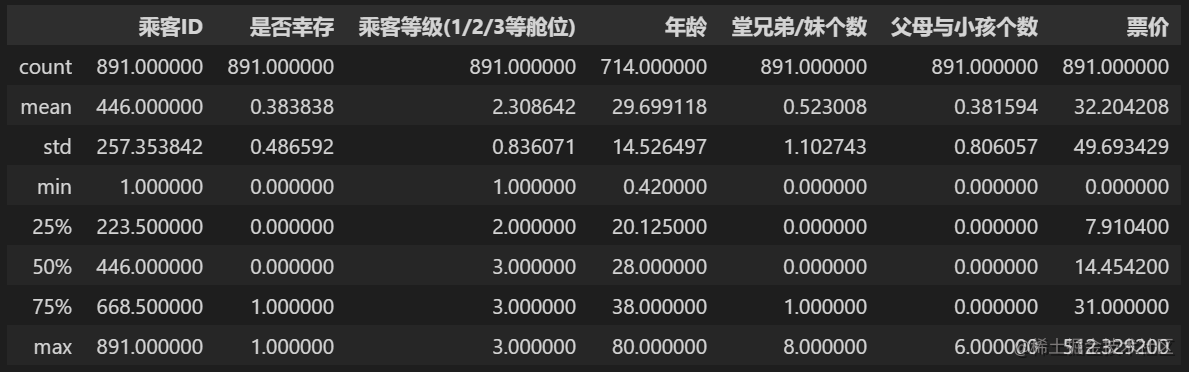```Python ''' 注意：方法describe()只统计（没有任何参数的情况下）数据类型（numeric-typed数据类型，例如int，float等）列的统计特征，并且会自动NaN值。 如果我们想查看所有列的统计特征（即包括非数据类型的列，例如object类型的列），就需要在describe()方法中添加参数（include = “all”） ''' data.describe(include='all')```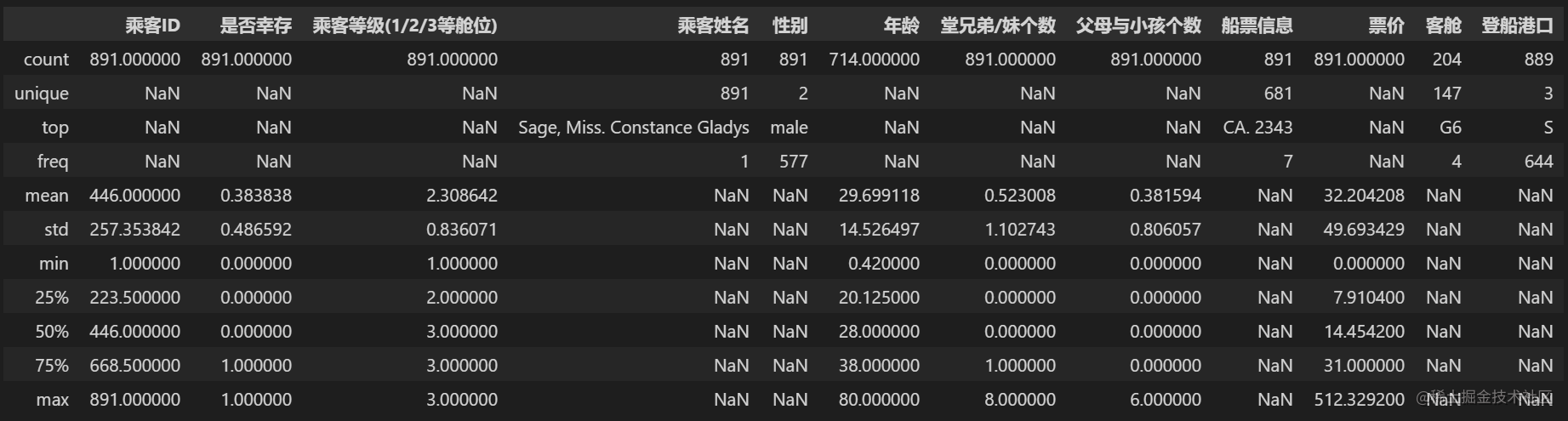```Python ''' 获取指定列的统计学特征 使用如下语句： dataframe[[‘column1’, ‘column2’, ‘column3’]].describe() ''' data[['乘客ID','年龄']].describe()```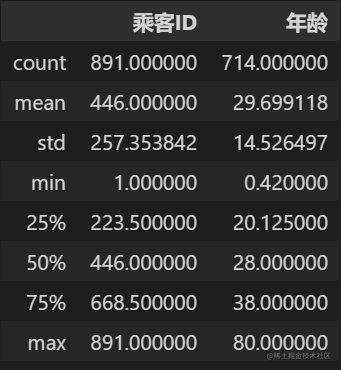```python ''' 使用方法info（）来查看dataframe的简介描述 使用如下语句： dataframe.info() 此方法打印有关dataframe的信息，包括索引dtype和列、非空值和内存使用情况。 ''' data.info()```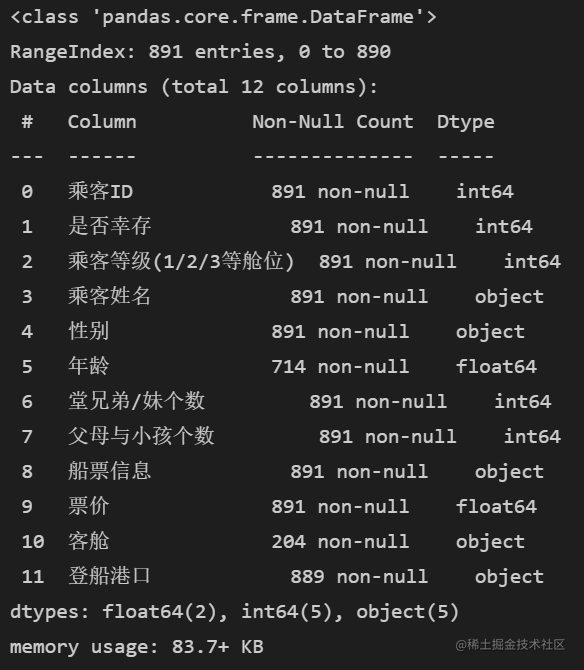```python ''' 查看数据的行数及列数 通过属性shape，获取数据集的（行数，列数） ''' print(data.shape)```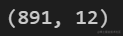```python ''' 是否包含null ''' print(data.isnull())```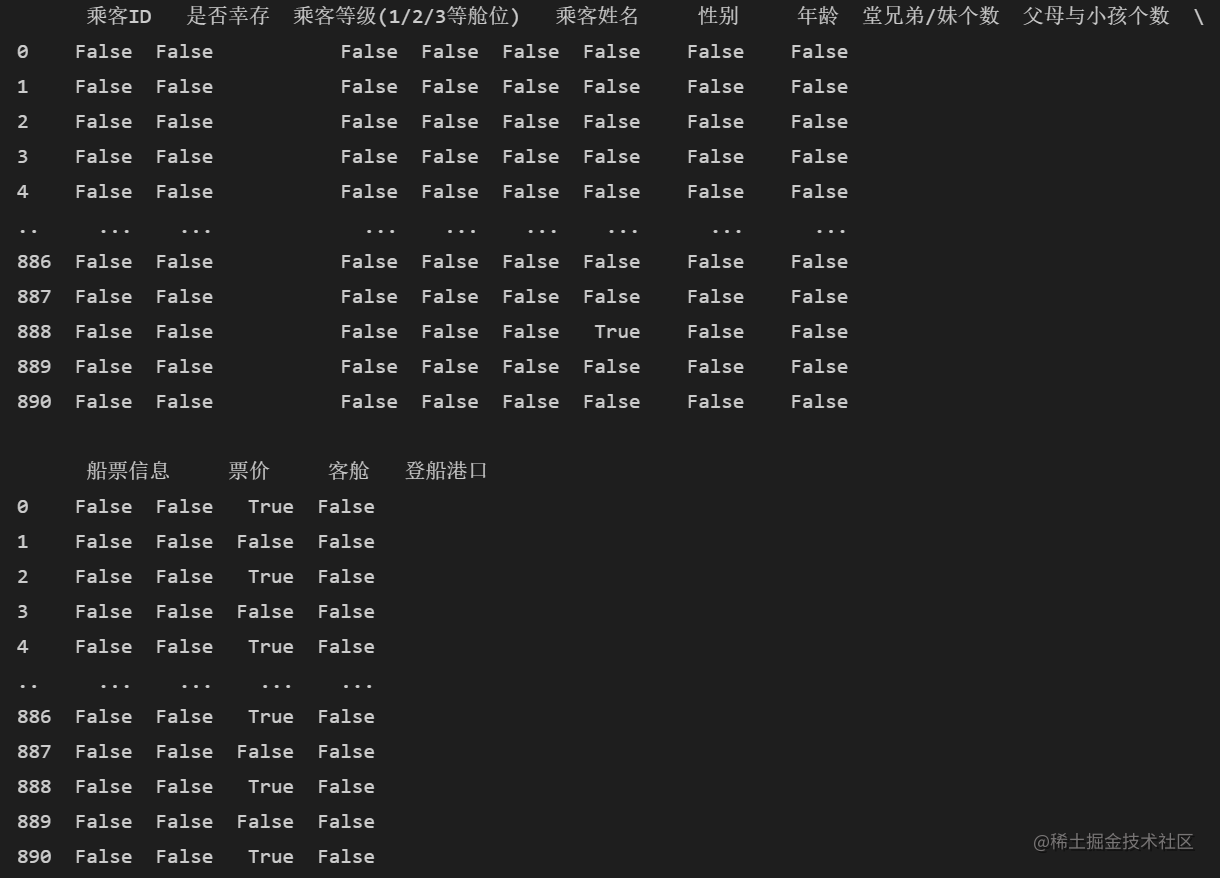```python

# 写入代码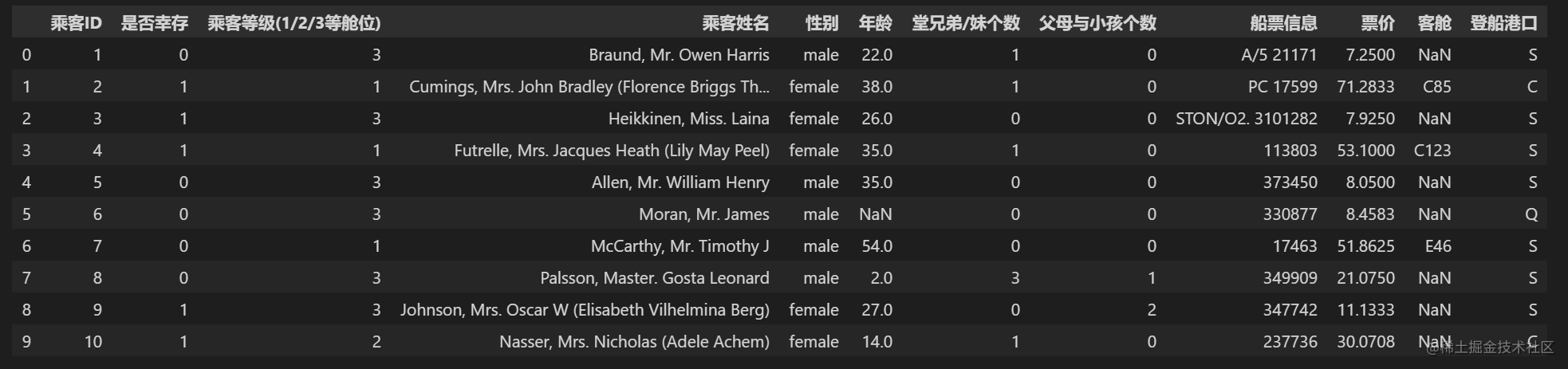```python

# 写入代码

data.tail(15) ```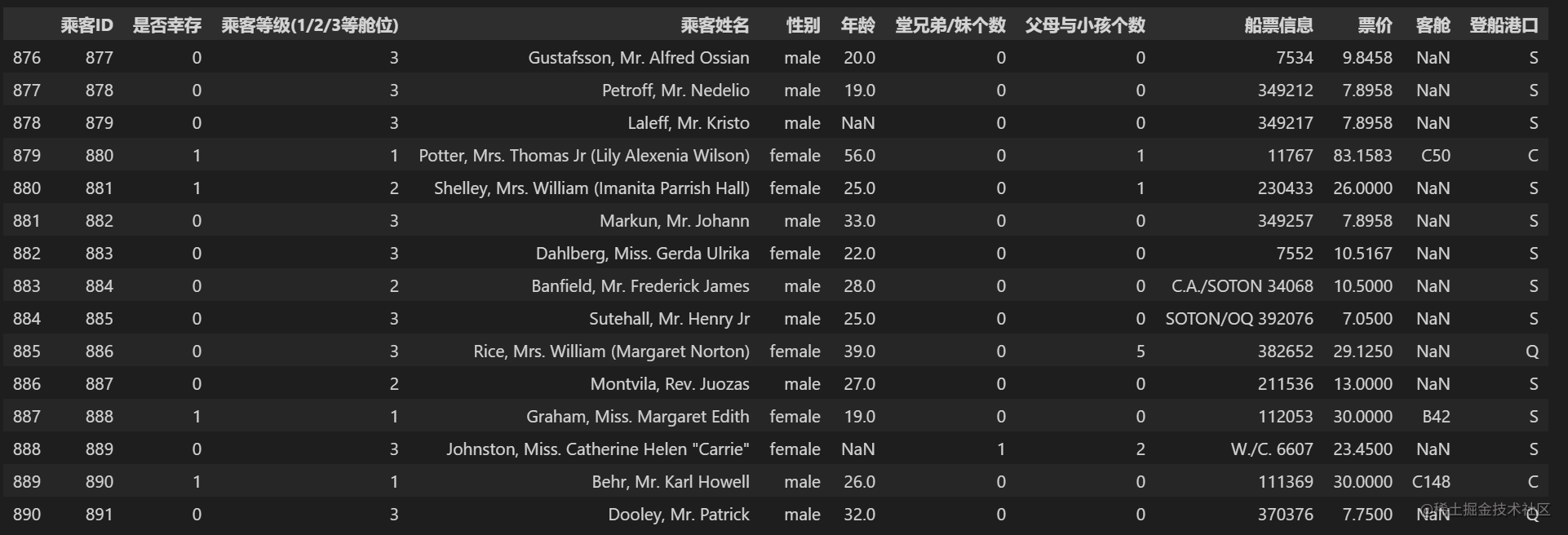```python

# 写入代码

''' 1、判断数值是否为空，可以用pd.isna,pd.isnull,np.isnan； 2、判断字符串是否为空，可以用pd.isna,pd.isnull； 3、判断时间是否为空，可以用pd.isna,pd.isnull，np.isnat； 4、判断转换类型后的字符串，空值也转换成了字符串nan，所以不能用常规方法判断了，直接判断字符串是否相等即可。 ''' data.isnull().head(20)

# data.isna()

```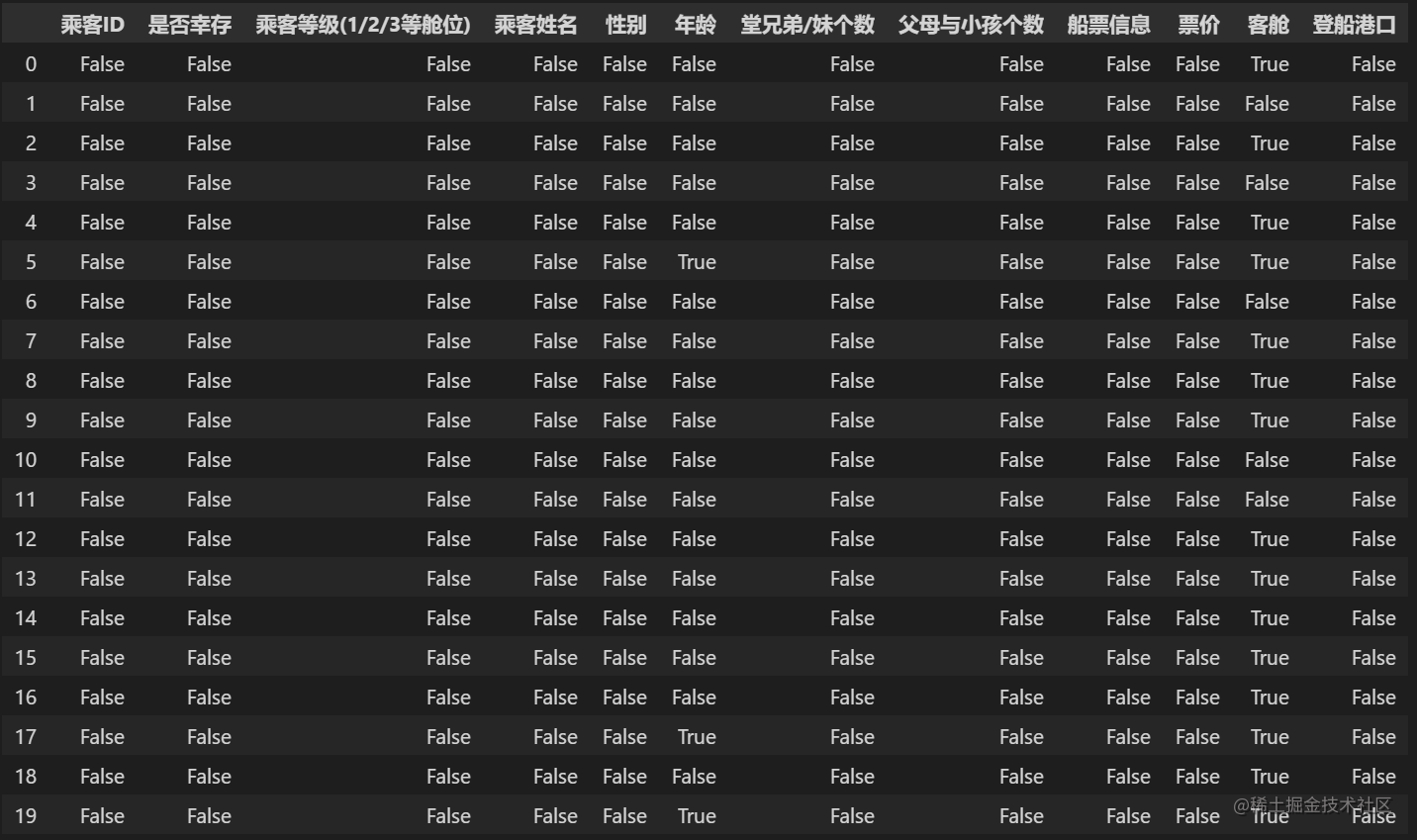【总结】上面的操作都是数据分析中对于数据本身的观察

```python

# 注意：不同的操作系统保存下来可能会有乱码。大家可以加入encoding='GBK' 或者 encoding = 'utf-8'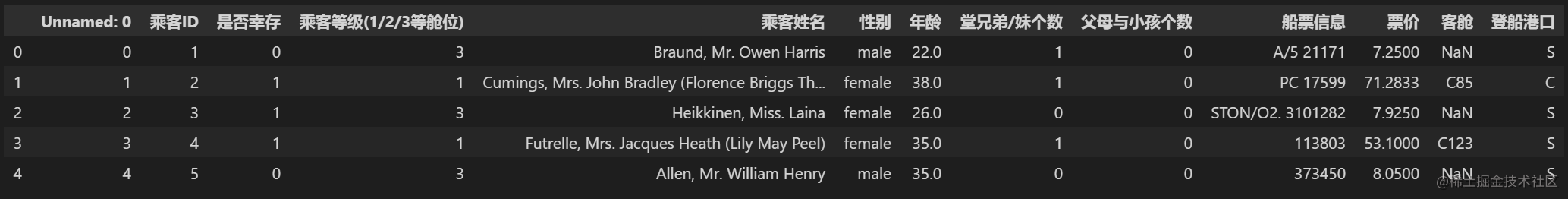【总结】数据的加载以及入门，接下来就要接触数据本身的运算，我们将主要掌握numpy和pandas在工作和项目场景的运用。

## 2 Pandas基础

### 1.4 知道你的数据叫什么

```python import numpy as np import pandas as pd```

```python

# 写入代码

''' 维数 名称 描述 1 Series 带标签的一维同构数组 2 DataFrame 带标签的，大小可变的，二维异构表格

# 为什么有多个数据结构？

Pandas 数据结构就像是低维数据的容器。比如，DataFrame 是 Series 的容器，Series 则是标量的容器。使用这种方式，可以在容器中以字典的形式插入或删除对象。 ''' sdata = {'Ohio': 35000, 'Texas': 71000, 'Oregon': 16000, 'Utah': 5000} example_1 = pd.Series(sdata) print(example_1) data = {'state': ['Ohio', 'Ohio', 'Ohio', 'Nevada', 'Nevada', 'Nevada'], 'year': [2000, 2001, 2002, 2001, 2002, 2003],'pop': [1.5, 1.7, 3.6, 2.4, 2.9, 3.2]} example_2 = pd.DataFrame(data) print(example_2) ```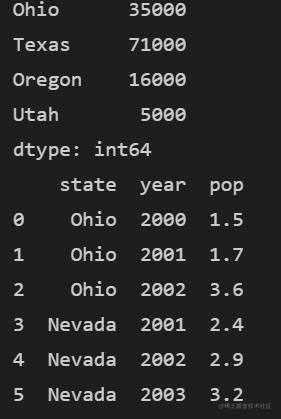```python

```python

# print(list(data))

data.columns ```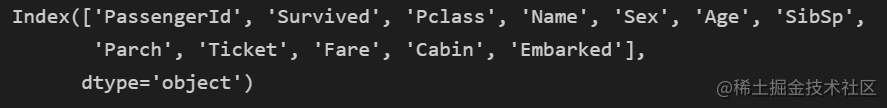```python

data['Cabin']

# 写入代码

data.Cabin ```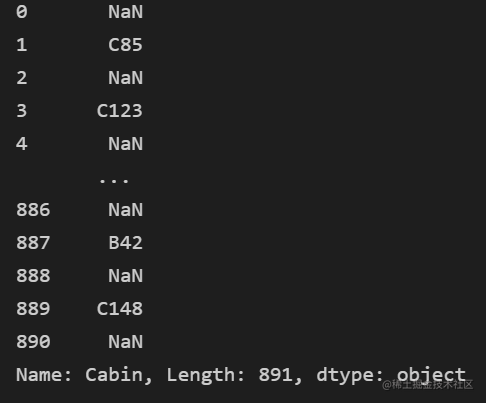```python

# 写入代码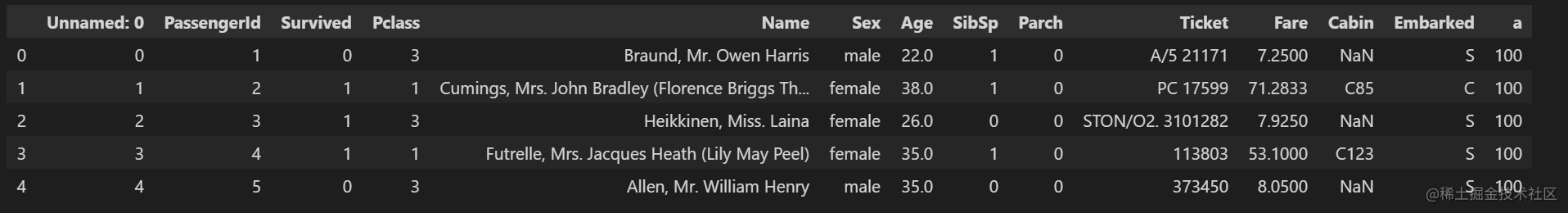```python

# 写入代码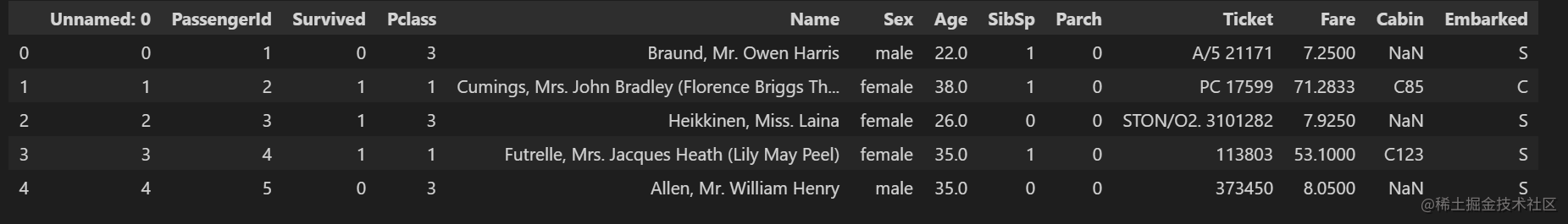【思考】还有其他的删除多余的列的方式吗？

```python

```python

# 写入代码

''' DataFrame.drop(labels=None, axis=0, index=None, columns=None, level=None, inplace=False, errors='raise')

```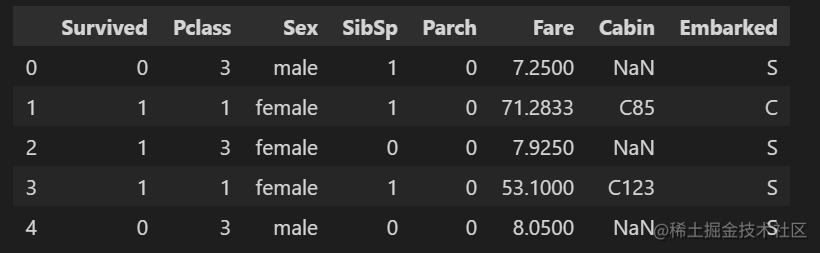【思考】对比任务五和任务六，是不是使用了不一样的方法(函数)，如果使用一样的函数如何完成上面的不同的要求呢？

【思考回答】 如果想要完全的删除你的数据结构，使用inplace=True，因为使用inplace就将原数据覆盖了，所以这里只是单纯的隐藏

```python

# 写入代码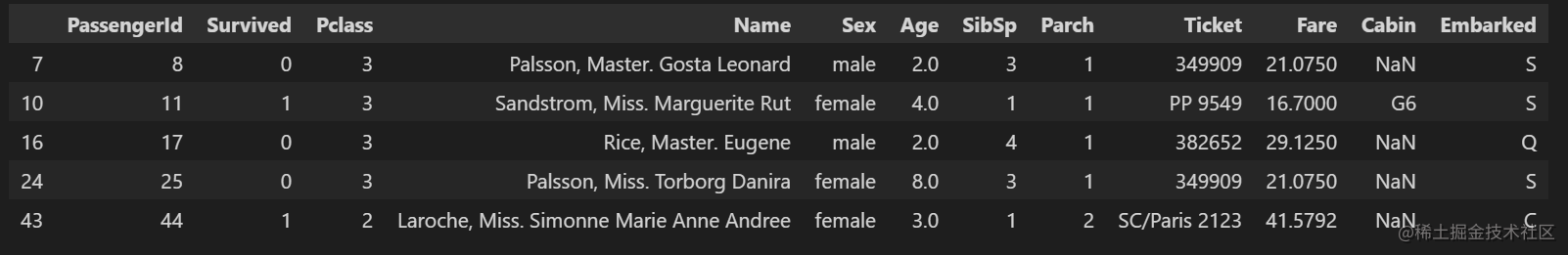```python

# 写入代码

midage=data[(data['Age']>10) & (data['Age']<50)] #此时的索引已经不连续了，需要重置索引 midage.head(100) ```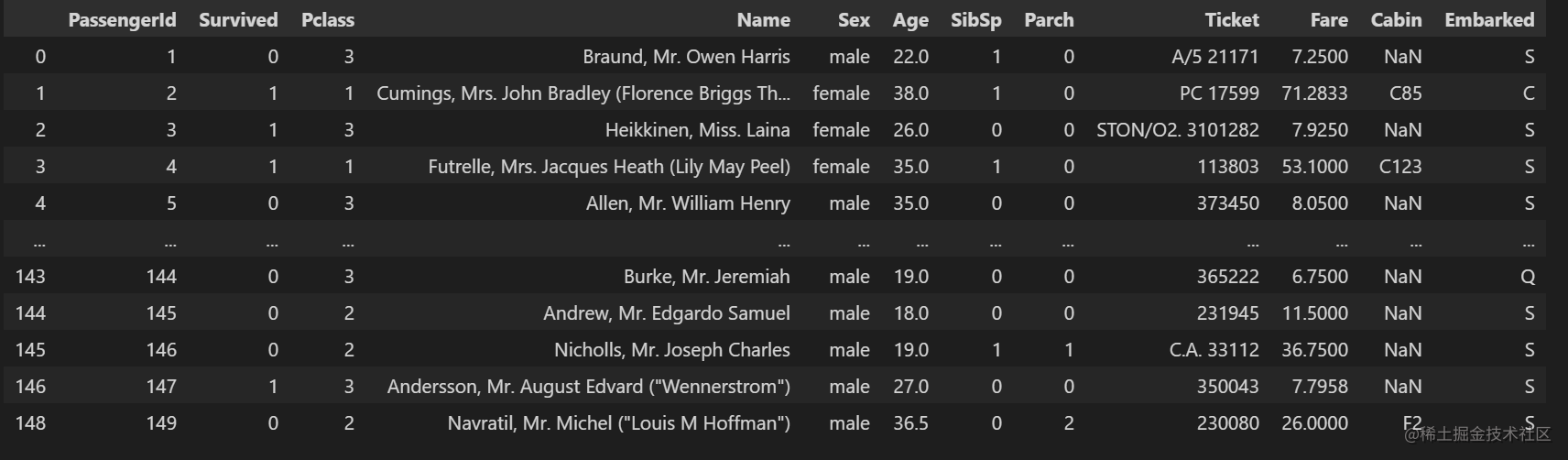【提示】了解pandas的条件筛选方式以及如何使用交集和并集操作

```python

# 写入代码

''' 此时DataFrame或Series类型的数据不再是连续的索引，可以使用reset_index()重置索引。

''' midage = midage.reset_index(drop=True) midage.head(100) midage.loc[,['Pclass','Sex']] ``````python

# 写入代码

midage.loc[[100,105,108],['Pclass','Name','Sex']]

# midage.loc[[100,105,108],'Pclass':'Sex'] #相同写法

```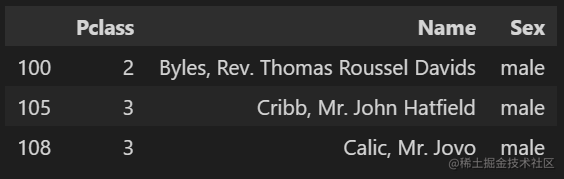```python

# 写入代码

midage.iloc[[100,105,108],[2,3,4]] ```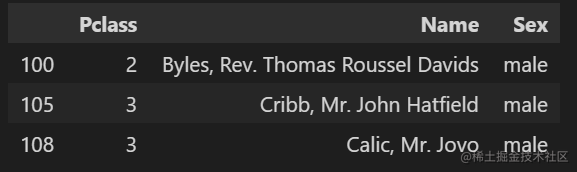【思考】对比`iloc``loc`的异同

• loc函数主要基于行标签和列标签（x_label、y_label）进行索引：使用loc函数，索引的是字符串，前后都要取，是属于“前闭后闭”的情况
• iloc函数主要基于行索引和列索引（index，columns） 都是从 0 开始：而且，iloc函数索引的数据是int整型，因此是Python默认的前闭后开。注意只能说int型，也就是数字，输入字符的话是会报错的。（注意索引都是默认从0开始的），而且传入的必须是一个列表

```python

# 加载所需的库

import numpy as np import pandas as pd

# 载入之前保存的train_chinese.csv数据，关于泰坦尼克号的任务，我们就使用这个数据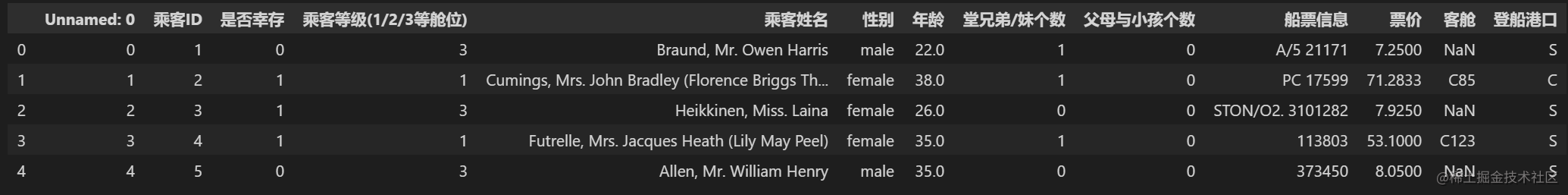```python

# 自己构建一个都为数字的DataFrame数据

''' 我们举了一个例子 pd.DataFrame() ：创建一个DataFrame对象 np.arange(8).reshape((2, 4)) : 生成一个二维数组（2*4）,第一列：0，1，2，3 第二列：4，5，6，7 index=[2，1] ：DataFrame 对象的索引列 columns=['d', 'a', 'b', 'c'] ：DataFrame 对象的索引行 ''' data1=np.arange(8).reshape((2,4))

# print(data)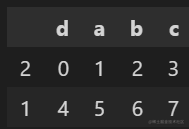【代码解析】

pd.DataFrame() ：创建一个DataFrame对象

np.arange(8).reshape((2, 4)) : 生成一个二维数组（2*4）,第一列：0，1，2，3 第二列：4，5，6，7

index=['2, 1] ：DataFrame 对象的索引列

columns=['d', 'a', 'b', 'c'] ：DataFrame 对象的索引行

【问题】：大多数时候我们都是想根据列的值来排序,所以将你构建的DataFrame中的数据根据某一列，升序排列

```python

# 回答代码

''' 升序排列是把数据从小到大进行排列，而降序排列是把数据从大到小进行排列 DataFrame.sort_values(by, ascending=True, inplace=Flase )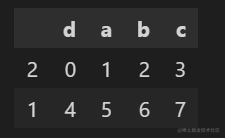【总结】下面将不同的排序方式做一个总结

1.让行索引升序排序

```python

# 代码

''' axis=0代表往跨行（down)，而axis=1代表跨列（across) 使用0值表示沿着每一列或行标签/索引值向下执行方法 使用1值表示沿着每一行或者列标签横向执行对应的方法 ''' df.sort_index(axis=0,ascending=True).head(2) ```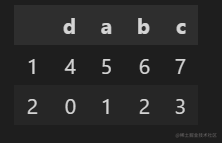axis的理解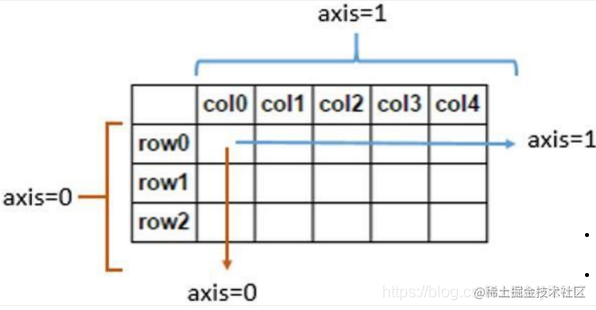2.让列索引升序排序

```python

# 代码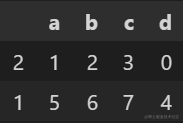3.让列索引降序排序

```python

# 代码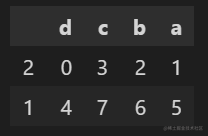4.让任选两列数据同时降序排序

```python

# 代码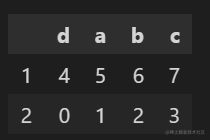```python

# 代码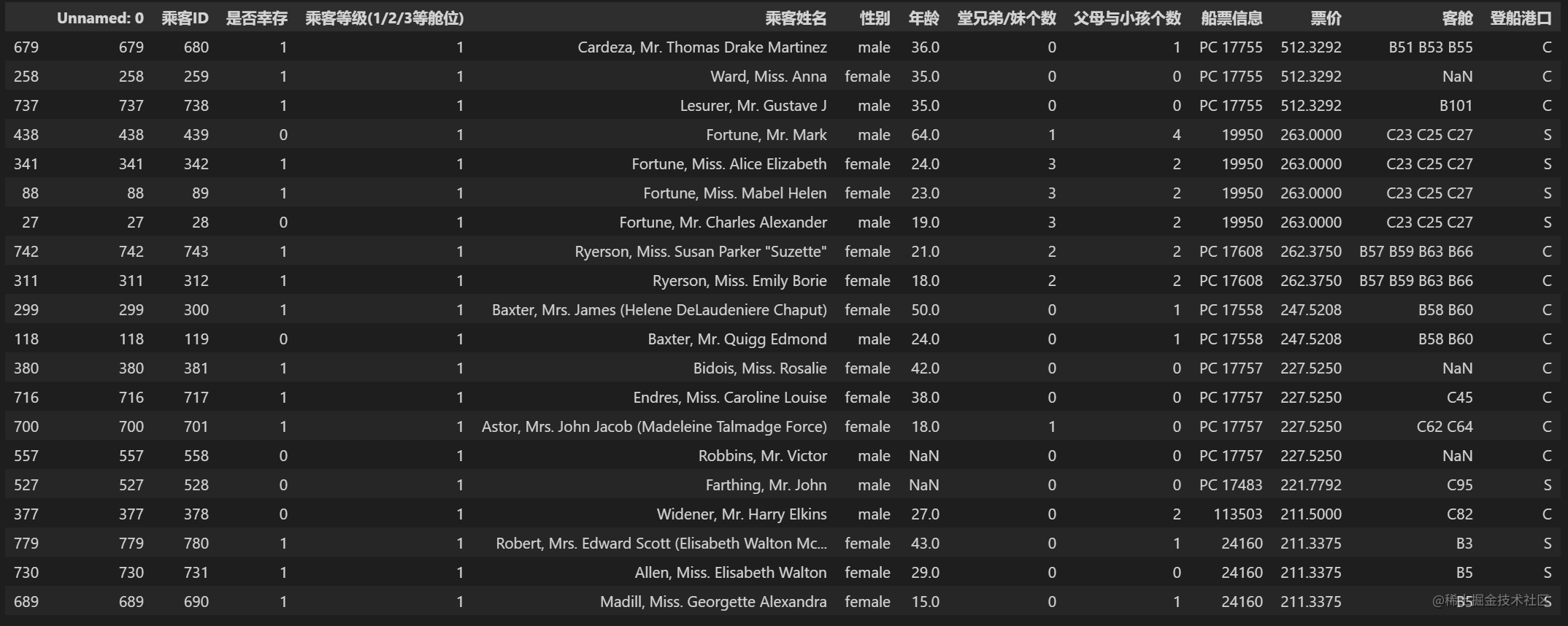【思考】排序后，如果我们仅仅关注年龄和票价两列。根据常识我知道发现票价越高的应该客舱越好，所以我们会明显看出，票价前20的乘客中存活的有14人，这是相当高的一个比例，那么我们后面是不是可以进一步分析一下票价和存活之间的关系，年龄和存活之间的关系呢？当你开始发现数据之间的关系了，数据分析就开始了。

```python d = {'A': [3, 6, 6, 7, 9], 'B': [2, 5, 8, 0, 0]} df = pd.DataFrame(data=d) print('排序前:\n', df) ''' 排序前: A B 0 3 2 1 6 5 2 6 8 3 7 0 4 9 0 ''' res = df.sort_values(by=['A', 'B'], ascending=[False, True]) print('按照A列B列的值排序:\n', res) ''' 按照A列B列的值排序: A B 4 9 0 3 7 0 1 6 5 2 6 8 0 3 2 '''``` 多列排序首先按照by[]列表和ascending[]列表中的对应顺序和对应逻辑进行排序，首先by列表的第一个列标签根据其在ascending列表的对应逻辑进行排序，其次再用by列表第二个列标签及其ascending列表的对应逻辑进行排序，以此类推。

```python

# 代码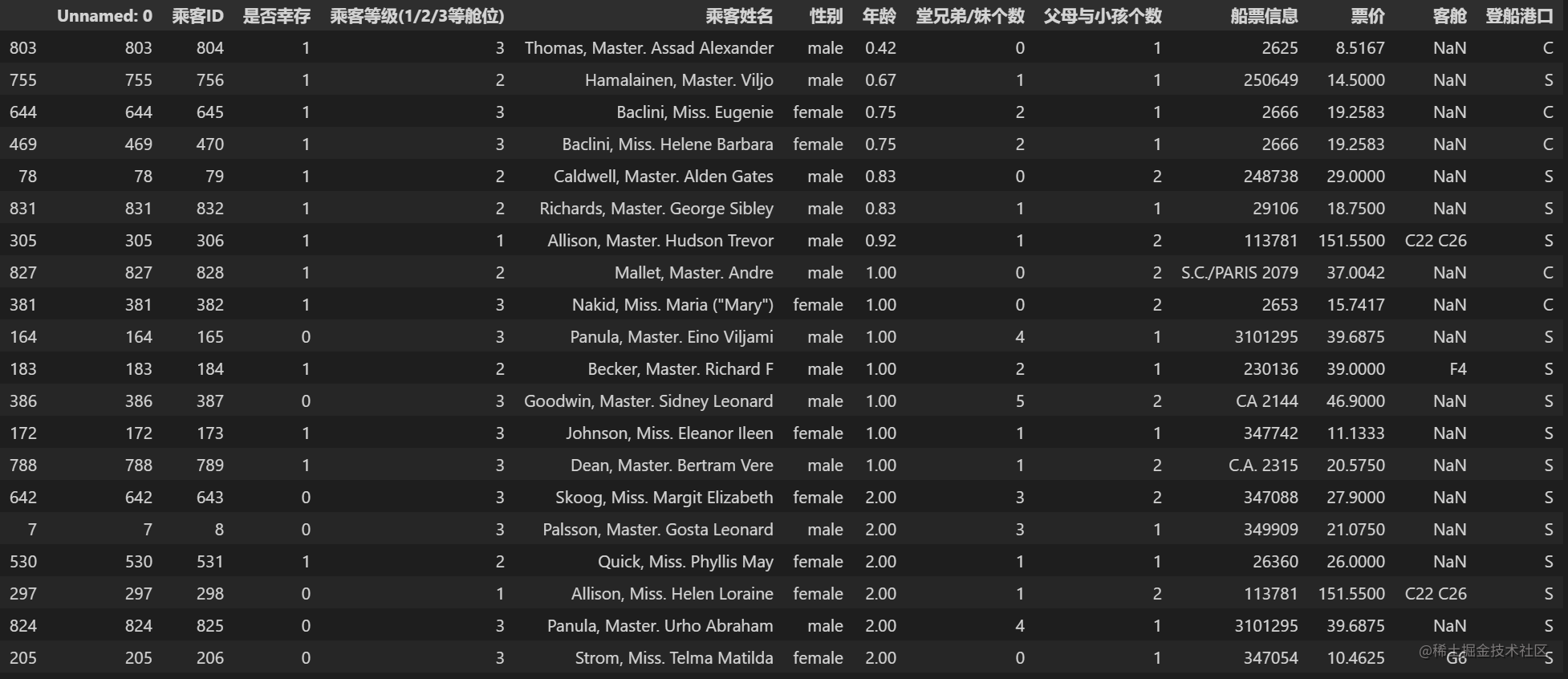```python

# 代码

frame1_a = pd.DataFrame(np.arange(9.).reshape(3, 3), columns=['a', 'b', 'c'], index=['one', 'two', 'three']) frame1_b = pd.DataFrame(np.arange(12.).reshape(4, 3), columns=['a', 'e', 'c'], index=['first', 'one', 'two', 'second']) print(frame1_a.head()) print(frame1_b.head()) ```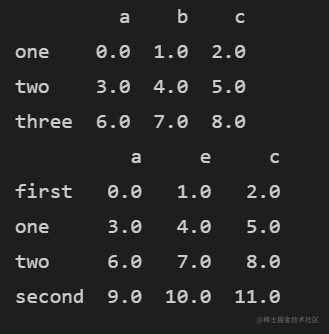```python

# 代码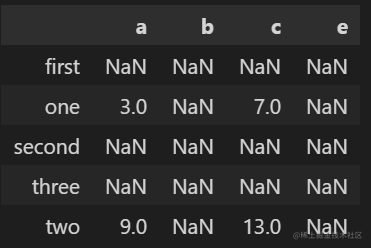# 代码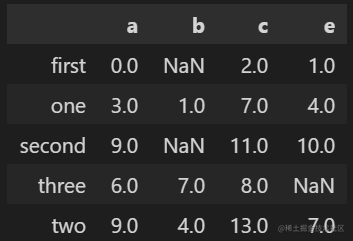【提醒】两个DataFrame相加后，会返回一个新的DataFrame，对应的行和列的值会相加，没有对应的会变成空值NaN。

```python

max(result)

``` 10

```python

# 代码

frame2 = pd.DataFrame([[1.4, np.nan], [7.1, -4.5], [np.nan, np.nan], [0.75, -1.3] ], index=['a', 'b', 'c', 'd'], columns=['one', 'two']) frame2 ```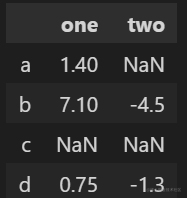```python

# 代码

frame2.describe() ```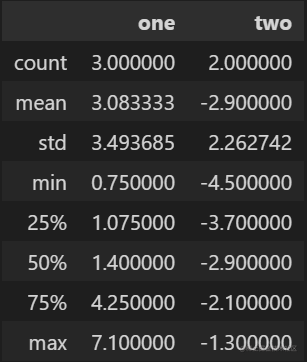#### 1.6.6 任务六：分别看看泰坦尼克号数据集中 票价、父母子女 这列数据的基本统计数据，你能发现什么？

```python ''' 看看泰坦尼克号数据集中 票价 这列数据的基本统计数据 '''

# 代码

data['票价'].describe() ```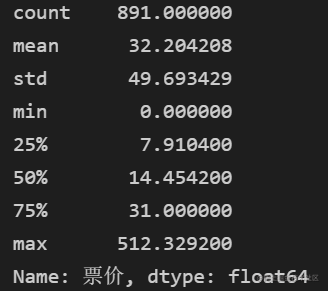【总结】本节中我们通过Pandas的一些内置函数对数据进行了初步统计查看，这个过程最重要的不是大家得掌握这些函数，而是看懂从这些函数出来的数据，构建自己的数据分析思维，这也是第一章最重要的点，希望大家学完第一章能对数据有个基本认识，了解自己在做什么，为什么这么做，后面的章节我们将开始对数据进行清洗，进一步分析。

「其他文章」